Courses

# Reduction of a Force and Couple System Mechanical Engineering Notes | EduRev

## Mechanical Engineering : Reduction of a Force and Couple System Mechanical Engineering Notes | EduRev

The document Reduction of a Force and Couple System Mechanical Engineering Notes | EduRev is a part of the Mechanical Engineering Course Engineering Mechanics - Notes, Videos, MCQs & PPTs.
All you need of Mechanical Engineering at this link: Mechanical Engineering

Further Reduction of a Force and Couple System

(Section 4.9)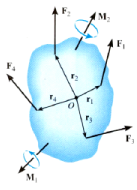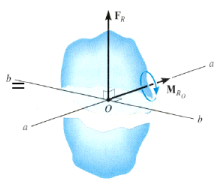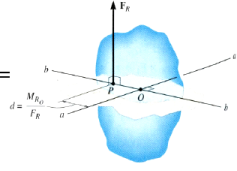If FR and MRO are perpendicular to each other, then the system can be further reduced to a single force, FR , by simply moving FR from O to P.

In three special cases, concurrent, coplanar, and parallel systems of forces, the system can always be reduced to a single force.

Example #1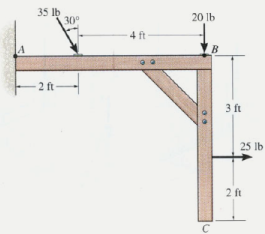Given: A 2­D force and couple system as shown.

Find: The equivalent resultant force and couple moment acting at A and then the equivalent single force location along the beam AB.

Plan:

1. Sum all the x and y components of the forces to find FRA.
2. Find and sum all the moments resulting from moving each force to A.
3. Shift the FRA to a distance d such that d = MRA/FRy

Example #1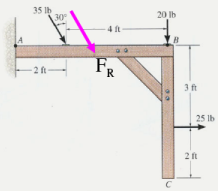+ → Σ FRx  =  25 + 35 sin 30°   = 42.5 lb

+  ↓ Σ FRy  =  20 + 35 cos 30°   = 50.31 lb

+  MRA   =  35 cos30° (2)  + 20(6)  – 25(3) = 105.6  lb∙ft

FR = ( 42.52 + 50.312 )1/2 = 65.9 lb

θ = tan-­1 ( 50.31/42.5) = 49.8 °

The equivalent single force FR can be located on the beam AB at a distance d measured from A.

d = MRA/FRy = 105.6/50.31 = 2.10 ft.

Example #2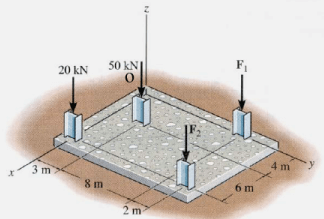Given: The building slab has four columns. F1 and F2 = 0.

Find:    The equivalent resultant force and couple moment at the origin O. Also find the location (x,y) of the single equivalent resultant force.

Plan:

1. Find FRO = ∑Fi = FRzo k
2. Find MRO = ∑ (ri × Fi) = MRxO i + MRyO j
3. The location of the single equivalent resultant force is given as x = ­MRyO/FRzO and y = MRxO/FRzO

Example #2

FRO = {­50 k – 20 k} = {­70 k} kN

MRO = (10 i) × (­20 k) + (4 i + 3 j)x(­50 k)

= {200 j + 200 j – 150 i} kN∙m

= {­150 i + 400 j } kN∙m

The location of the single equivalent resultant force is given as,

x = ­MRyo/FRzo = ­400/(­70) = 5.71 m

y = MRxo/FRzo = (­150)/(­70) = 2.14 m

Offer running on EduRev: Apply code STAYHOME200 to get INR 200 off on our premium plan EduRev Infinity!

## Engineering Mechanics - Notes, Videos, MCQs & PPTs

30 videos|72 docs|65 tests

,

,

,

,

,

,

,

,

,

,

,

,

,

,

,

,

,

,

,

,

,

;